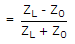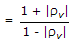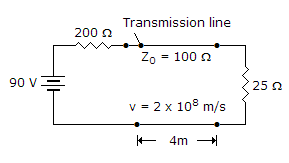# Electronics and Communication Engineering - Microwave Communication

### Exercise :: Microwave Communication - Section 4

16.

A 75 ohm line is first short terminated and minima locations are noted. Then the short is replaced by resistive load and minima location are again noted. If minima location are not altered and VSWR is 3, the value of resistive load is

 A. 25 Ω B. 50 Ω C. 225 Ω D. 250 Ω

Explanation:

rvand VSWR.

17.

The word 'LORAN' means

 A. long range navigation B. long range TV transmission C. long range cable transmission D. either (b) or (c)

Explanation:

No answer description available for this question. Let us discuss.

18.

In the given figure forward voltage wave has a magnitudeA. 90 V B. 60 V C. 30 V D. 10 V

Explanation:

No answer description available for this question. Let us discuss.

19.

The duty cycle of a radar transmitter is equal to

 A. (PRF) (pulse width) B. (PRF)/(pulse width) C. (pulse width)/(PRF) D. (pulse width) + (PRF)

Explanation:

No answer description available for this question. Let us discuss.

20.

In a klystron amplifier the bunching effect

 A. converts velocity modulation into current modulation of beam B. converts current modulation into velocity modulation of beam C. both (c) and (b) D. neither (a) nor (b)

Explanation:

A Klystron is a vacuum tube used for generation/amplification of microwaves.

An electron beam is produced by oxide coated indirectly heated cathode and is focussed and accelerated by focussing electrode.

This beam is transmitted through a glass tube. The input cavity where the beam enters the glass tube is called buncher.

As electrons move ahead they see an accelerating field for half cycle and retarding field for the other half cycle.

Therefore, some electrons are accelerated and some are retarded. This process is called velocity modulation.

The velocity modulation causes bunching of electrons. This bunching effect converts velocity modulation into density modulation of beam.

The input is fed at buncher cavity and output is taken at catcher cavity.

In a two cavity klystron only buncher and catcher cavity are used. In multi cavity klystron one or more intermediate cavities are also used.

The features of a multicavity klystron are :

1. Frequency range - 0.25 GHz to 100 GHz

2. Power output - 10 kW to several hundred kW

3. Power gain - 60 dB (nominal value)